• 在OIOIOI中，我们简化了一下向量的存储方式及运算法则，我们定义向量为起点为(0,0)(0,0)(0,0)的一条有方向的线段。由于我们在考虑向量时只考虑其大小与方向，一般不考虑具体位置，故我们将向量平移至坐标...
关于向量
高中数学必修$4$说:

几何向量是线性空间中有大小与方向的量。

放图理解一下：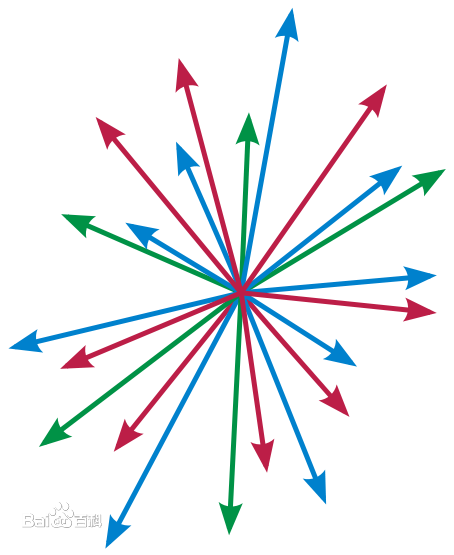如上图所示，向量可以形象的用一根箭头表示。箭头所指代表向量的方向，线段的长度代表向量的大小。
在$OI$中，我们简化了一下向量的存储方式及运算法则，我们定义向量为起点为$(0,0)$的一条有方向的线段。由于我们在考虑向量时只考虑其大小与方向，一般不考虑具体位置，故我们将向量平移至坐标系原点处以简化运算。
所以，在之后的讲解中，我们默认向量和点已经被存在了一个结构体里面（向量和点记录的东西都是一个坐标）：
struct Point{
double x,y;  //注意这个double
};

如果想分清楚具体是点还是向量，那么只需要在结构体后加入一句：
typedef Point Vector;

这样我们就可以只开一个结构体而做到存储两种量。
tips:如果您觉得只用Point即可，那么请将后文的Vector全部视为Point（这样写出来也没有任何问题）
向量的运算法则（具体原理请查阅数学必修$4$）
前置：模长
$|A|$表示向量$A$的长度，为一个数。
double Length(const Vector &p){
return sqrt(p.x*p.x+p.y*p.y);
}

前置：向量的方向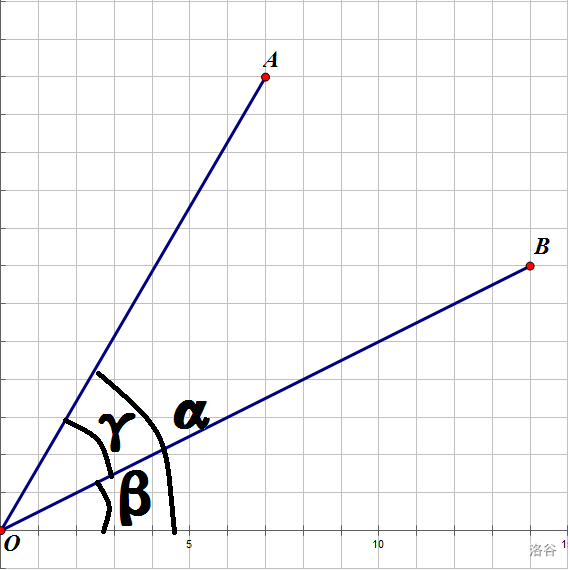（请暂且忽略$B$以及$\beta和\gamma$，后面要用）
我们设向量$A$为$(x,y)$，那么
$cos(\alpha)=sin(90^。-\alpha)=\frac{x}{|A|}$
$sin(\alpha)=cos(90^。-\alpha)=\frac{y}{|A|}$
那么，我们定义向量$A$的方向为$w(cos(\alpha),sin(\alpha))。$
点±向量=点
//所有的重载运算符都定义在结构体外部，如果不重载运算符的话会很麻烦
Point operator + (const Point &p,const Vector &q){
return (Point){p.x+q.x,p.y+q.y};
}
Point operator - (const Point &p,const Vector &q){
return (Point){p.x-q.x,p.y-q.y};
}

点-点=向量
Vector operator - (const Point &p,const Point &q){
return (Vector){p.x-q.x,p.y-q.y};
}

友情提示：两个减的操作只需要写一个，如果实在看不顺眼，那么就讲其中一个减号改为另外一个不相冲突的符号。
点+点无意义
向量*常数=向量
Vector operator * (const Vector &p,const double &k){
//double很重要！！！
return (Vector){p.x*k,p.y*k};
}

向量/常数=向量
Vector operator / (const Vector &p,const double &k){
return (Vector){p.x/k,p.y/k};
}

向量±向量=向量
代码同点±向量
向量的点积
令向量$X=(x_1,x_2),Y=(y_1,y_2),$则有：
定义式：$X\cdot Y=|X||Y|cos(\gamma)$，其中$\gamma$为$X,Y$的夹角
但更通用的是这样一个式子：$X\cdot Y=|X||Y|cos(\gamma)=x_1y_1+x_2y_2$
double Dot (const Vector &p,const Vector &q){
return p.x*q.x+p.y*q.y;
}

几何意义：A在B所在方向的投影的模长和B的模长的乘积
tips:如果向量$A,B$的点积为$0$，则$A,B$垂直
证明如下：我们有上面讲到的向量的方向可知，
$cos(\alpha)=\frac{x_1}{|X|},sin(\alpha)=\frac{x_2}{|Y|}$
$cos(\beta)=\frac{y_1}{|X|},sin(\beta)=\frac{y_2}{|Y|}$
由$\gamma=\alpha-\beta$得：
$cos(\gamma)=cos(\alpha-\beta)$
由数学必修4上一个著名公式得：
$cos(\gamma)=cos(\alpha-\beta)=cos(\beta)cos(\alpha)+sin(\beta)sin(\alpha)$
$cos(\gamma)=\frac{x_1}{|X|}\times\frac{y_1}{|Y|}+\frac{x_2}{|X|}\times\frac{y_2}{|Y|}$
$cos(\gamma)=\frac{x_1y_1+x_2y_2}{|X||Y|}$
$X\cdot Y=|X||Y|cos(\gamma)=x_1y_1+x_2y_2$
向量的叉积
定义式：$X*Y=|X||Y|sin(\gamma)$
通用的式子：$X*Y=|X||Y|sin(\gamma)=x_1y_2-x_2y_1$
double Cross (const Vector &p,const Vector &q){
return p.x*q.y-q.x*p.y;
}

证明大体同上，需要用到另一个著名的式子：
$sin(α-β)=sin(α)cos(β)-cos(α)sin(β)$
几何意义：A转到B所夹平行四边形的有向面积
简单地解释一下：
在$A,B$不共线的情况下，如果$A$在$B$上面，那么它们的叉积就小于$0,$反之则大于$0$。
如果$A,B$共线，那么它们的叉积就等于$0$。
基础运算完整代码
struct Point{
double x,y;
};
typedef Point Vector;
Point operator + (const Point &p,const Vector &q){
return (Point){p.x+q.x,p.y+q.y};
}
Vector operator - (const Point &p,const Point &q){
return (Vector){p.x-q.x,p.y-q.y};
}
Vector operator * (const Vector &p,const double &k){
return (Vector){p.x*k,p.y*k};
}
Vector operator / (const Vector &p,const double &k){
return (Vector){p.x/k,p.y/k};
}
double Dot (const Vector &p,const Vector &q){
return p.x*q.x+p.y*q.y;
}
double Cross (const Vector &p,const Vector &q){
return p.x*q.y-q.x*p.y;
}
double Length(const Vector &p){
return sqrt(Dot(p,p));
}



展开全文• 1.1平面向量的加法运算 两个向量，； 向量满足四边形法则； 1.2平面向量的乘法运算 两个向量，； 向量乘表示为 ; 相比于向量加运算，向量乘运算要复杂点，很难看明白向量乘的几何意义； 将和用极坐标表示...
1.平面向量

1.1平面向量的加法运算

两个向量，；

向量满足四边形法则；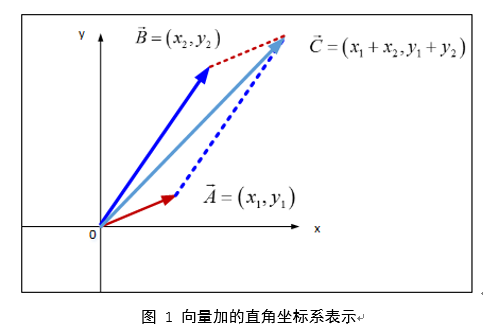1.2平面向量的乘法运算

两个向量，；

向量乘表示为

;

相比于向量加运算，向量乘运算要复杂点，很难看明白向量乘的几何意义；

将和用极坐标表示：

则向量乘法表示为：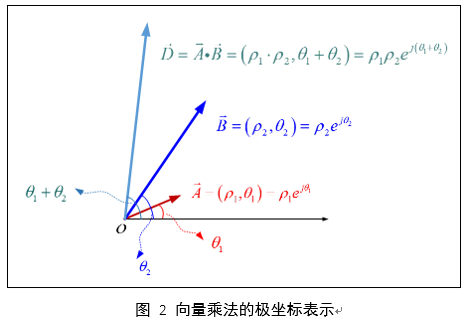2.空间向量

如果三个向量、、不共面，那么对空间任一向量，存在唯一的有序实数组，使的 。

模长；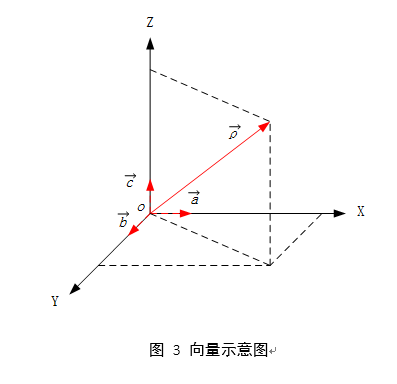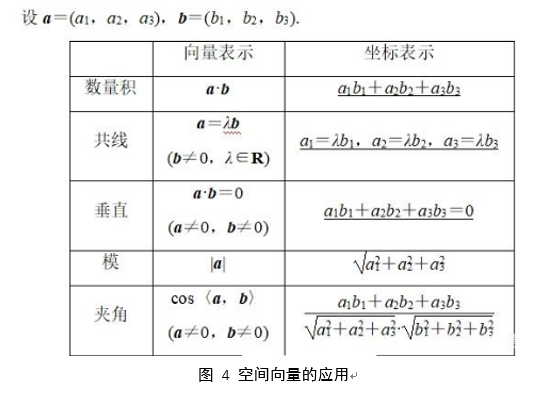展开全文• 基本运算 向量的加法 首尾相连法则(当然还有其他名字:平行四边形法则、三角形法则) 如图a⃗+b⃗\vec{a}+\vec{b}a+b就相当于将b⃗\vec{b}b的起点平移到a⃗\vec{a}a的终点(a⃗、b⃗\vec{a}、\vec{b}a、b以原点为起点)...
向量
有大小，有方向的量，记为$\vec{a}$
基本运算
向量的加法
首尾相连法则(当然还有其他名字:平行四边形法则、三角形法则)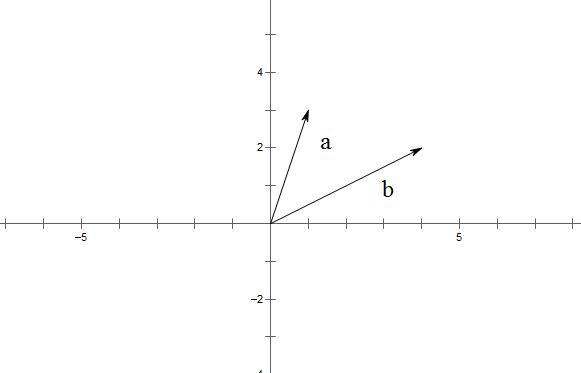如图$\vec{a}+\vec{b}$就相当于将$\vec{b}$的起点平移到$\vec{a}$的终点($\vec{a}、\vec{b}$以原点为起点)，得到$\vec{b'}$，就如下图: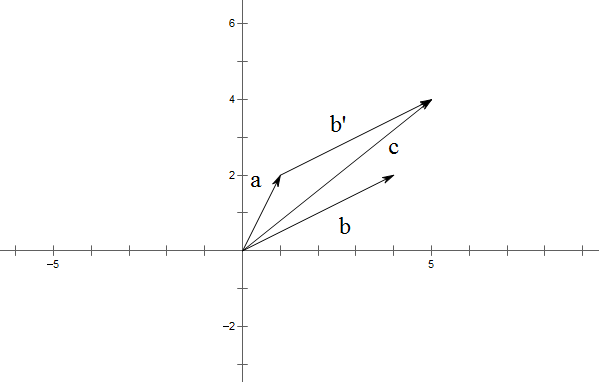$\vec{a}+\vec{b}=\vec{c}$，因为它们作用效果一样，这就是首尾相连法则，即由$\vec{a}$的起点，到$\vec{b'}$的终点。
向量的积
数量积
即一个向量与一个标量相乘，即数乘。
如下图: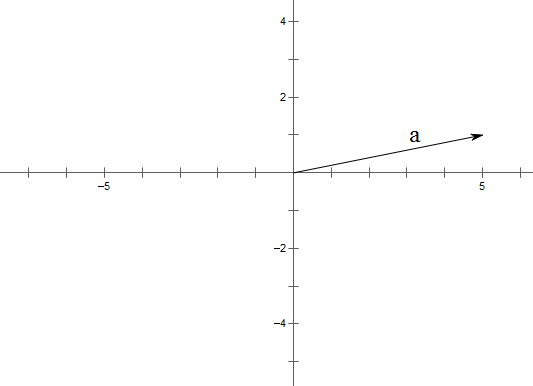代数表示为:$\lambda\vec{a}$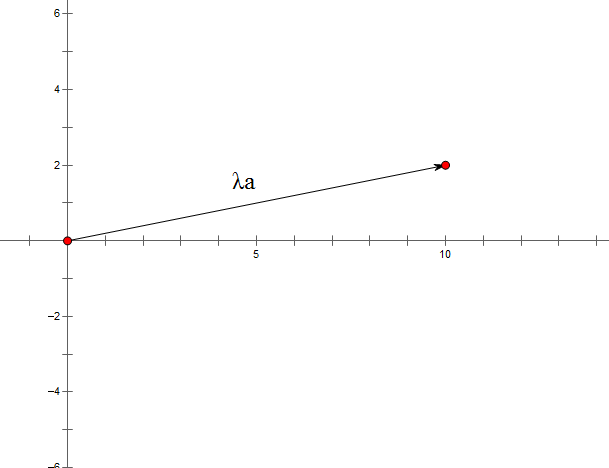内积
两个向量点乘，代数表示为:$\vec{a}\cdot \vec{b}=\mid\vec{a}\mid\mid\vec{b}\mid cos\theta$
意义为:$\vec{a}$的大小与$\vec{b}$在$\vec{a}$上的投影的大小的乘积
乘积为标量。
若$\vec{a}$为$(x_1,y_1)$,$\vec{b}$为$(x_2,y_2)$
则$\vec{a}\cdot \vec{b}=x_1*x_2+y_1*y_2$
具体证明略，详见$60$课时学高中数学。

外积
两个向量叉乘为外积，外积是一个向量。
$\vec{a}\times\vec{b}=\mid\vec{a}\mid\mid\vec{b}\mid sin\theta=x_1*y_2-x_2*y_1$
其中$\theta$表示$\vec{a}$沿着逆时针方向旋转到$\vec{b}$的度数。
这里其实有点问题，外积是一个向量，但运算结果是一个标量(我解决不了)，但是在计算几何中，用到的往往是关于与向量类似的运算，所以理解就好了。在做题中，我们仅仅只是用(伪外积)去求一个线段到另外一个线段，是如何旋转(度数严格小于$180°$)，从而确定线段是顺时针还是逆时针旋转，或者用来去掉重复的面积的。
这点应该要清楚理解。
而不是
$\mid\vec{a}\times\vec{b}\mid=\mid\vec{a}\mid\mid\vec{b}\mid sin\theta=x_1*y_2-x_2*y_1$
其中$\theta$表示$\vec{a}$与$\vec{b}$的夹角($0\le\theta\le 180°$)度数。
证明不知道。


展开全文• 向量的基本概念1. 线性运算1.1 加减法运算1.2 共线定理知识点结论总结2. 等比数列 1. 线性运算 1.1 加减法运算 向量加法的三角形法则：将第一个向量的终点与第二个向量的起点重合，以第一个向量的起点为起点，第二...
向量的基本概念1. 线性运算1.1 加减法运算1.2 共线定理知识点结论总结1.1 向量与三角形知识点2. 基本定理与坐标表示2.1 基本定理的应用知识点2.2 坐标运算知识点
1. 线性运算
1.1 加减法运算

向量加法的三角形法则：将第一个向量的终点与第二个向量的起点重合，以第一个向量的起点为起点，第二个向量的终点为终点的向量为和向量。
向量加法的平行四边形法则：若两个向量是从同一点出发的不共线向量，以这两个向量为领边作平行四边形，则以同一起点为起点的对角线所对应的向量为和向量。
向量减法的三角形法则：将两个向量的起点放在一起，以减向量的终点为起点，被减向量的终点为终点的向量为差向量。

1.2 共线定理
知识点

一般地，我们规定实数 $λ$ 与向量 $\vec{a}$ 的积是一个向量，这种运算叫做向量数乘，记作 $λ\vec{a}$，它的长度和方向规定如下：
（1）$|λ\vec{a}| = |λ||\vec{a}|$；
（2）当 $λ>0$ 时，$λ\vec{a}$ 与 $\vec{a}$ 的方向相同；当 $λ<0$ 时，$λ\vec{a}$ 与 $\vec{a}$ 的方向相反；当 $λ=0$ 时，$λ\vec{a}$ = $\vec{0}$；
根据实数与向量积的定义，有如下运算律：
若 $λ,μ$ 为实数，则：
（1）$λ(μ\vec{a}) = (λμ)\vec{a}$
（2）$(λ+μ)\vec{a} = λ\vec{a}+μ\vec{a}$
（2）$λ(\vec{a}+\vec{b}) = λ\vec{a}+λ\vec{b}$

向量 $\vec{a} (\vec{a} ≠ \vec{0})$ 与 $\vec{b}$ 共线，当且仅当存在唯一一个实数 $λ$ ，使得 $\vec{b} = λ\vec{a}$。
【注】向量共线定理的应用需要特别注意 $(\vec{a} ≠ \vec{0})$，否则，若 $\vec{a} = 0$ 且 $\vec{b} ≠ \vec{0}$，则不存在 $λ$ 使得 $\vec{b} = λ\vec{a}$；若 $\vec{a} = 0$ 且 $\vec{b} = \vec{0}$，则使得 $\vec{b} = λ\vec{a}$ 成立的实数 $λ$ 不唯一；

结论总结

$\overrightarrow{AB} = λ\overrightarrow{BC} \iff \overrightarrow{OC} = x\overrightarrow{OA} + y\overrightarrow{OB} (x+y=1)$。

【证明】（1）从左边往右边证明，由于 $\overrightarrow{AB} = λ\overrightarrow{BC}$，由向量减法可得 $\overrightarrow{OB} - \overrightarrow{OA} = λ(\overrightarrow{OC}-\overrightarrow{OB})$，整理可得 $\overrightarrow{OC} = (1+\dfrac{1}{λ})\overrightarrow{OB} - \dfrac{1}{λ}\overrightarrow{OA}$，令 $x=-\dfrac{1}{λ}$，$y=1+\dfrac{1}{λ}$，可得 $x+y=1$，得证。
（2）从右边往左边证明，因为 $\overrightarrow{OC} = x\overrightarrow{OA} + y\overrightarrow{OB} (x+y=1)$，所以 $\overrightarrow{OC} = x\overrightarrow{OA} + (1-x)\overrightarrow{OB}$，整理得 $x(\overrightarrow{OA}-\overrightarrow{OB}) = \overrightarrow{OC} - \overrightarrow{OB}$，则 $-x\overrightarrow{AB} = \overrightarrow{BC}$，令 $λ=-\dfrac{1}{x}$，可得 $\overrightarrow{AB} = λ\overrightarrow{BC}$，得证。
【注】若点 $O$ 与 $A$，$B$，$C$三点不共线，$A$，$B$，$C$三点的位置可以任意互换，但当$A$，$B$，$C$ 三点的相对位置不同时，系数 $x$，$y$ 的正负会随之改变，可以利用向量加法的平行四边形法则进行解释。
1.1 向量与三角形
知识点

若向量 $\vec{a}$，$\vec{b}$ 不平行，则 $\vec{a}$，$\vec{b}$，$\vec{a}+\vec{b}$，$\vec{a}-\vec{b}$，可以构成一个平行四边形的两邻边和两对角线。
根据三角形三边之间的关系，我们可以得到 $|\vec{a}|$，$|\vec{b}|$，$|\vec{a}|+|\vec{b}|$，$|\vec{a}|-|\vec{b}|$ 之间的关系：
（1）利用三角形三边之间的关系，可以得到 $||\vec{a}| - |\vec{b}|| ≤ |\vec{a}|±|\vec{b}| ≤ |\vec{a}| + |\vec{b}|$
（2）$|\vec{a} + \vec{b}|^2 + |\vec{a} - \vec{b}|^2 = 2(|\vec{a}|^2 + |\vec{b}|^2)$。

2. 基本定理与坐标表示
2.1 基本定理的应用
知识点

如果 $\vec{e_1}$，$\vec{e_2}$ 是同一个平面内的两个不共线的非零向量，那么对于这一平面内的任意向量 $\vec{a}$，有且只有一对实数 $λ$，$μ$，使 $\vec{a} = λ\vec{e_1} + μ\vec{e_2}$，其中，不共线的向量 $\vec{e_1}$，$\vec{e_2}$叫做表示这一平面内所有向量的一组基底。

2.2 坐标运算
知识点

向量加法、减法、数乘向量
设 $\vec{a} = (x_1, y_1)$，$\vec{b} = (x_2, y_2)$，则 $\vec{a} + \vec{b} = (x_1+x_2, y_1+y_2)$，$\vec{a} - \vec{b} = (x_1-x_2, y_1-y_2)$，$λ\vec{a} = (λx_1, λy_1)$。
向量坐标的求法
（1）若向量的起点是坐标原点，则终点坐标即为向量的坐标。
（2）设 $A (x_1,y_1)$，$B(x_2,y_2)$，则 $\overrightarrow{AB} = (x_2-x_1, y_2-y_1)$。
平面向量共线
设 $\vec{a}=(x_1,y_1)$，$\vec{b}=(x_2,y_2)$，其中 $\vec{b} ≠ \vec{0}$，则 $\vec{a} ∥ \vec{b} \iff x_1y_2-x_2y_1=0$。


展开全文数学
• 1、平面向量的基本概念(1)既有大小又有方向的量叫向量，只有大小没有方向的量叫数量。(注意物理学中的矢量与向量的区别)(2)共线向量、相等向量、零向量的概念：两个向量的有向线段所在的直线互相平行或重合，则这两...
• 背景介绍今天我们学习使用numpy的内置数学运算方法和基本的算术运算符两种方式对数组进行数学运算的学习，内容涉及到线性代数的向量矩阵的基本运算知识(不熟悉的童鞋回头自己补一下哈)，接下来开始：入门示例以下为...
• 1.1 导数运算法则 条件：f(x),g(x)f(x), g(x)f(x),g(x)都是可导。 结论： [f(x)±g(x)]′=f′(x)+g′(x)[f(x)\pm g(x)]^{&#x27;}=f^{&#x27;}(x)+g^{&#x27;}(x)[f(x)±g(x)]′=f′(x)+g′(x) [f(x)g(x...
• 向量的基本运算法则，构成了整个线性代数的基础。在物理学中，特别是求系统平衡时，经常碰到这类问题，给定若干矢量，这些矢量和能与某特定矢量平衡吗？这就需要研究向量组的线性组合，本节给出低维空间中的情况，...线性代数 矩阵
• 这篇文章记录了在学习中对线性代数一些基本概念或定理理解，同时发掘一下其中想法以及与其他知识之间联系。1 概述与定义1.1 内积空间概述内积空间是线性代数理论中重要组成部分，而想要理解它，最好先问自己...
• 向量微积分基本定理问题引入向量积分梯度、散度与旋度散度与旋度的运算法则积分公式的向量形式应用举例例题1例题2练习例子，弧长积分求面积，注意极坐标弧长微分公式对坐标曲线积分回顾格林公式回顾 ...算法
• 空间向量的基本概念1.空间向量的概念：定义：在空间，我们把具有大小和方向的量叫做向量。模长：向量的大小叫做向量的模，a的模长记作￨a￨备注：文中加粗的小写字母均代表向量。2.空间向量的运算：运算法则：与平面...
• 一、问题引入——格林公式、高斯公式、斯托克斯公式 ...3. 散度与旋度的运算法则 四、积分公式的向量形式 1. 微积分基本公式（定积分、曲线积分） 2. 通量计算 3. 环流量计算 ...
• 向量求导与标量求导法则不同的是，向量的求导还要注意结果的排法问题。注意排法是因为当一个目标函数是多个成分相加时，如果不注意排法可能导致有些结果是行，有些是列，无法继续进行运算。我总结的向量求导的基本...
• ## 李宏毅 线性代数 向量和矩阵

千次阅读 多人点赞 2019-07-23 08:26:23
1. 向量 1.1 基本定义   根据高中所学，向量就是一组数字，用符号vvv来表示。...  向量的相加可以使用平行四边形法则或者三角形法则。 1.4 向量集合   向量集合可以包括无穷多个向量。如...
• 1．高二数学平面向量基本概念：向量的定义、向量的模、零向量、单位向量、相反向量、共线向量、相等向量。2．高二数学平面向量加法与减法的代数运算：(1)若a=(x1，y1)，b=(x2，y2)则ab=(x1+x2，y1+y2)．向量加法与...
• 向量几何 ... 2016年3月30日   1 目标：研究物体位置、移动等空间相关关系 2 原理：使用带有方向量表示空间中物体，并研究其...3 方法：基本运算 加法：平等四边形法则。 平行、与数乘法：l=kv，向量l与v平数学 几何 矩阵
• 文章目录1.1 向量1.1.1 物理中的向量1.1.2 向量与复数1.1.3 数学中的向量1.1.4 大小和方向1.2 向量的加减和数乘1.2.1 加法1.2.2 数乘1.2.3基本运算法则1.3.1 等比混合1.3.2 非等比混合1.3.3 线性组合1.3.4 线性相关...
• 变量名最多不超过63个字符; ? 变量名区分大小写;... 2.2.2 矩阵常规运算 在matlab中,矩阵常规运算即是指按矩阵 运算法则进行运算,主要有以下几种: + 加法 - 减法 * 乘法 ^......2.1 基本语法结构 2.1.2...
• ## 向量空间初步

千次阅读 2015-10-26 11:03:02
向量空间是由向量，以及他们加法、乘法作为运算符作为基本元素组成集合。 空间往往有一些特殊属性。比如向量空间定义了向量计算一些限制，比如封闭率（集合元素运算的结果仍在集合中）、结合率等等。 再比如...
• 一、向量的基本内容 向量即矢量，既有方向又有大小，比如物理量位移、速度、力 向量a⃗=b⃗\vec{a}=\vec{b}a=b意味着： a⃗，b⃗\vec{a}，\vec{b}a，b方向相同，大小相等 1.向量的大小 用向量的模表示 模等于1的...
• 1.1与向量有关的基本概念（基础） 向量：记住是一个矢量，带方向 向量的模：向量的大小 向量的坐标及坐标表示：向量在三个坐标轴上的投影叫做向量的坐标，记为： 单位向量： 向量的方向余弦： 1.2向量运算与性质 ...
• 基本概念 向量：既有大小又有方向的量，又称矢量 向量的大小叫向量的长度（模） 在线性代数中只研究自由向量 相等：向量a与b大小相等、方向相同；...使用平行四边形法则和三角形法则进行向量的加减 数数学 线性代数
• 矩阵的基本运算 常见矩阵 导数 向量求导 导数运算法则 导数法则 常用函数及其导数 Logistic 函数 Softmax 函数 向量 标量（Scalar） 实数 向量 (Vector) n个实数组成的有序数组，称为n维向量。...
• 《高等代数与符号运算》是高等代数经典理论与计算机符号运算的有机结合，是数值分析、最优化方法、微分方程、控制论与矩阵分析、数学模型等数学分支及工程学科重要应用工具，《高等代数与符号运算》是为适应现代...
• 内容概述 ...接着，讲了矩阵方程Ax=bA\boldsymbol x =\boldsymbol bAx=b一些基本性质，例如解存在性，AxA\boldsymbol xAx如何计算，以及AxA\boldsymbol xAx这一矩阵-向量的运算法则。 矩阵方...线性代数 机器学习 矩阵
• 四则运算法则 复合函数求导法则 神经网络激活函数导函数求解 高阶导数 导数与函数单调性 极值定理 导数与函数凹凸性 一元函数泰勒展开 线性代数基础 向量与其运算 行向量和列向量 向量加减、数乘、内积、转置 向量...人工智能 机器学习
• 1函数的单调性2函数的奇偶性3函数在某处的导数的几何意义4几种常见函数的导数5导数的运算法则6求函数的极值7分数指数幂8根式的性质9有理数指数幂的运算性质10对数公式11常见的函数图像12同角三角函数的基本关系式13...
• 矩阵运算法则 单位矩阵 矩阵逆 矩阵转置 矩阵，向量 基本概念 主要讲了些基本概念，可由图直观理解。 矩阵 向量 矩阵运算 矩阵加，减法（需要两个矩阵规模完全一致） 实数和矩阵运算（会对...吴恩达
• 这门课重要性不言而言，它是解释线性代数来源以及它可以怎么用重点，而在学校里面，我们只能够学到它定义和计算方式。 1 向量是什么？ 1.1问题 向量是什么？...（向量就这两种基本运算...